# 7 Graphs

1. A directed graph whose nodes (vertices) are of type alpha can be represented as
```type graph(alpha) == set(alpha X alpha);
```
Each pair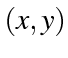in the set represents an edge from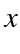to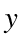.

What does the following function do?:
```f: graph(alpha) -> set(alpha);
f(G) <= if G=empty
then empty
else let ((a,b),G1) == choose(G)
in (a & (b & empty)) U f(G1);
```

2. Write a function:

```outdegree: alpha X graph(alpha) -> num;
```
which returns the out-degree of a particular vertex in the graph.
3. Write a function `nexts` which, given a vertex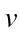and a graph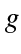finds the set of vertices that are at the end of an out-going edge from.

4. Given a function
```cfp: alpha -> graph(alpha) -> set(list(alpha));
```
which is such that `cfp x g` is the set of all cycle-free paths from `x` in `g`, write a function
```       reachable: alpha X graph(alpha) -> set(alpha);
```
such that `reachable(x, g)` is the set of all vertices reachable from `x` in `g`. (You may need to define some extra functions.)
5. Using `cfp`, write a function for finding the length of the shortest path between two vertices in a graph. (You may need to define some extra functions. )

s.danicic@gold.ac.uk
Sebastian Danicic BSc MSc PhD (Reader in Computer Science)
Dept of Computing, Goldsmiths, University of London, London SE14 6NW
Last updated 2011-03-15